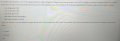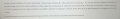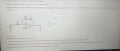# About the amplifier gain

#### Chalielogan

Joined Apr 11, 2022
8At the first picture, I can find until Power gain. But however, I am bit confused with rms value. The input current has rms value. Then should I have to apply rms value whilst finding voltage gain, power gain? I apply rms value to output current whilst calculating current gain. And with next two following pictures, can you give me some tip and solution please?

#### Papabravo

Joined Feb 24, 2006
18,964
RMS (Root Mean Square) values are intended to be used for power calculations since they correctly estimate the average power consumed by an AC waveform.

$$V_{gain}\;=\;20 log \left( \cfrac{V_o}{V_i}\right)$$
$$I_{gain}\;=\;20 log \left( \cfrac{I_o}{I_i}\right)$$

but power gain is different

$$P_{gain}\;=\;10log\left(\cfrac{P_o}{P_i}\right)$$

Input and output power are computed using RMS values for voltage and current.

#### Irving

Joined Jan 30, 2016
2,979
For Q2 try drawing the equivalent circuit to help you visualise it. From that it will become clear what voltages you have... Post your thinking here...

For Q3 you need to write the transfer equation, eg Vo = F(Vi) as a function of R1, R2, C and frequency. Do you know the formula for the impedance of a capacitor as a function of frequency? As a hint, the transfer equation for an inverting opamp without the capacitor is Vo = -(R2/R1) . Vi

Again post your thinking here...

#### WBahn

Joined Mar 31, 2012
26,831
It's not a good idea to post multiple questions in a single thread -- the usual result is chaos as different posts are talking about different problems and everyone gets confused over which problem they are making a comment on.

Also, the general expectation is that you show your best attempt at solving your problem. We then will try to help you identify where you are going wrong and guide you toward a good path that will allow you to solve the problem that you were assigned and that you are seeking credit for.